# 自然语言处理 13：形式语言理论和有限状态自动机

## 墨尔本大学 COMP90042 课程笔记

Posted by YEY on April 15, 2020

# Lecture 13 形式语言理论和有限状态自动机

## 1. 形式语言理论

• 语言模型
• 隐马尔可夫模型
• 循环神经网络

### 1.2 形式语言理论

• 一种语言 $=$ 字符串 (strings) 的集合
• 一个字符串 $=$ 来自一个有限 字母集 (alphabet)元素 (element) 所组成的序列
• 字母集可以视为 词典 (vocabulary)
• 元素可以视为 单词 (words)

### 1.3 动机

• 正则语言 (Regular Language)
• 上下文无关语言 (Context Free Language)

### 1.4 例子

• 二进制串（Binary strings）以 0 开头，以 1 结尾
• $\{01, 001, 011, 0001, \dots\}$ ⭕️
• $\{1, 0, 00, 11, 100, \dots\}$ ❌
• 来自字母集 $\{a, b\}$ 的偶数长度的序列
• $\{aa, ab, ba, bb, aaaa, \dots\}$ ⭕️
• $\{aaa, aba, bbb, \dots\}$ ❌
• 以 wh- 类型的单词作为开头，问号 ？结尾的英文句子
• $\{\textit{what }?, \textit{where my pants }?, \dots\}$ ⭕️

### 1.5 除了从属问题之外的问题

• 从属问题（Membership）
• 某个字符串是否属于某种语言？是/否
• 记分（Scoring）
• 具有记分等级的从属关系
• 某个字符串在多大程度上可以被接受？（语言模型）
• 转导（Transduction）
• 将一个字符串转变为另一个字符串（词干提取 stemming）

### 1.6 概览

• 正则语言（Regular languages）
• 有限状态接收器 & 转换器（Finite state acceptors & transducers）
• 单词形态学建模（Modelling word morphology）

## 2. 正则语言

### 2.1 正则语言

• 正则语言（Regular language）：语言中最简单的类别。
• 任何 正则表达式（regular expression）都是一种正则语言。
• 描述了什么样的字符串是该语言的一部分
• 正式地，一个正则表达式包含以下运算：
• 从字母集中抽样得到的符号：$\Sigma$
• 空字符串：$\varepsilon$
• 两个正则表达式的连接：$RS$
• 两个正则表达式的交替：$R\mid S$
• 星号表示出现 0 次或者重复多次：$R^*$
• 圆括号定义运算的有效范围：$()$

### 2.2 正则语言的例子

• 二进制串（Binary strings）以 0 开头，以 1 结尾
• $0 \,(0\mid 1)^* 1$
• 来自字母集 $\{a, b\}$ 的偶数长度的序列
• $((aa)\mid (ab)\mid (ba)\mid (bb))^*$
• 以 wh- 类型的单词作为开头，问号 ？结尾的英文句子
• $((what)\mid (where)\mid (why)\mid (which)\mid (whose)\mid (whom))\Sigma^*?$

### 2.3 正则语言的性质

• 封闭（Closure）：如果我们对正则语言 L1 和 L2 进行合并，得到的结果仍然是正则语言吗？如果是，那么我们将该运算称为 封闭运算（closed operation）
• 在以下运算中，正则语言是封闭的：
• 连接（concatenation）求并（union）：来自封闭的定义。
• 求交（intersection）：在正则语言 L1 和 L2 中都合法的字符串。
• 求反（negation）：不在正则语言 L 中的字符串。
• 这种性质非常万金油，可以有不同的正则语言来描述语言的不同性质，然后将它们合并起来。
• 可以使用相同的核心算法来完成这些事。

## 3. 有限状态接收器（FSA）

### 3.1 有限状态接收器

• 正则表达式定义了一个正则语言。
• 但是，它并没有给出一种算法来检查某个字符串是否属于该语言。
• 有限状态接收器（Finite state acceptors，FSA）给出了检查某个成员（字符串）是否属于某个正则语言的算法。
• FSA 包含：
• 输入符号的字母集：$\Sigma$
• 状态集合：$Q$
• 起始状态：$q_0\in Q$
• 最终状态：$F\subseteq Q$
• 转移函数：符号和状态 $\to$ 下一个状态
• 如果存在一条从 $q_0$ 到最终状态的 路径（path），并且转移函数与路径上的每个符号都匹配，则接受该字符串。
• Djisktra 最短路径算法，$O(V\log V +E)$

### 3.2 FSA 的例子

• 输入字母集：$\{a,b\}$
• 状态：$\{q_0,q_1\}$
• 起始状态：$q_0$
• 最终状态：$\{q_1\}$
• 转移函数：$\{(q_0,a) \to q_0, \;(q_0,b) \to q_1, \;(q_1,b) \to q_1\}$
• 注意：如果在 $q_1$ 状态下应用 $a$ 符号会导致失败。
• 接受 $a^*bb^*$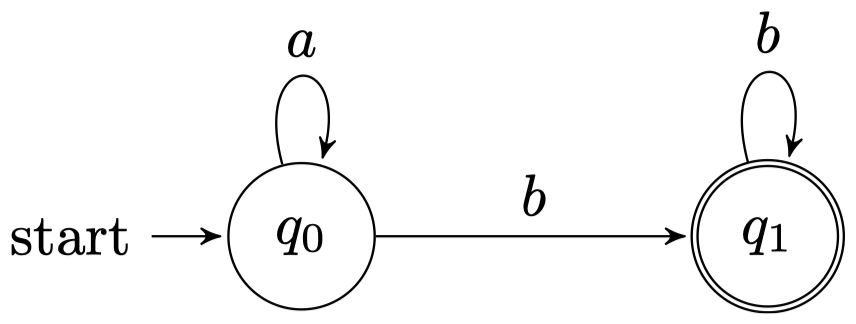### 3.3 派生形态

• $\textit{grace} \to \textit{graceful} \to \textit{gracefully}$
• $\textit{grace} \to \textit{disgrace} \to \textit{disgracefully}$
• $\textit{allure} \to \textit{alluring} \to \textit{alluringly}$
• $\textit{allure} \to *\textit{allureful}$
• $\textit{allure} \to *\textit{disallure}$

### 3.4 FSA 用于单词形态学

• 希望接受有效形式，拒绝无效形式（带 $*$ 标记）
• 泛化到其他单词，例如：表现类似 $\textit{grace}$ 或者 $\textit{allure}$ 的名词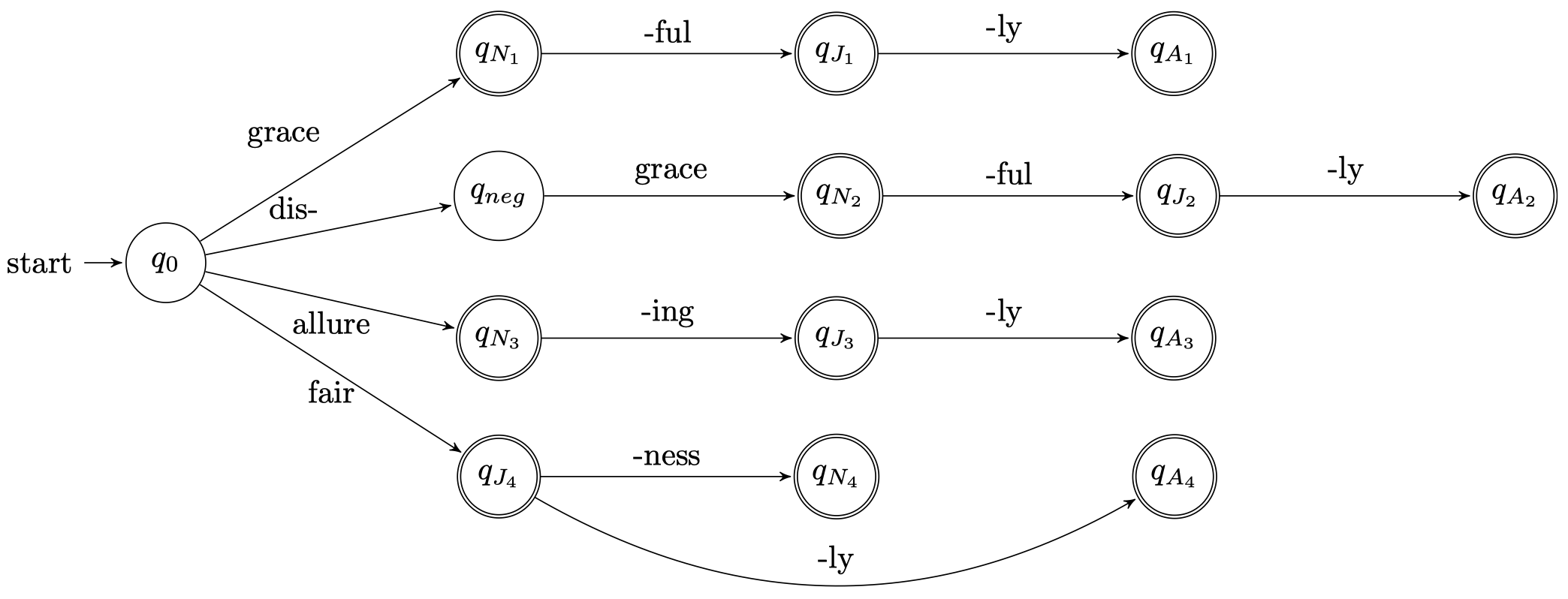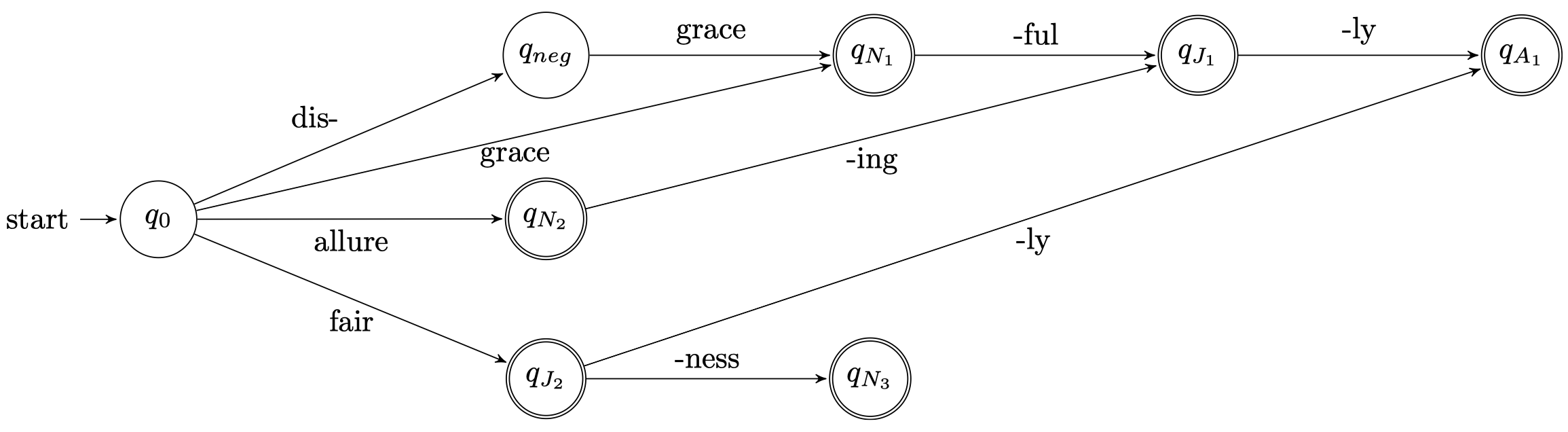## 4. 加权 FSA

### 4.1 加权 FSA

• 有些单词比其他单词更有可能被接受：
• $\textit{fishful}$ vs. $\textit{disgracelyful}$
虽然二者都不是合法的英文单词，但是，从某种程度上，我们可以认为 $\textit{fishful}$ 要比 $\textit{disgracelyful}$ 更有可能作为一个合法英文单词，因为其从字面上更容易理解一些。
• $\textit{musicky}$ vs. $\textit{writey}$
同理，$\textit{musicky}$ 要比 $\textit{writey}$ 更有可能成为一个形容词。所以，有些单词作为合法单词的可能性要高于其他一些单词，语言和单词并不仅仅是二元类别的。
• 关于可接受程度的记分方式 —— 加权 FSA
• 状态集合：$Q$
• 输入符号的字母集：$\Sigma$
• 起始状态加权函数：$\lambda: Q \to \mathbb R$
• 最终状态加权函数：$\rho: Q \to \mathbb R$
• 转移函数：$\delta: (Q,\Sigma, Q) \to \mathbb R$

### 4.2 WFSA 最短路径

$\lambda(t_0)+\sum_{i=1}^{N}\delta(t_i)+\rho(t_N)$

### 4.3 N-gram 语言模型作为 WFSA

$P(w_1,\dots,w_M)\approx \prod_{m=1}^{M}P_n(w_m\mid w_{m-1},\dots,w_{m-n+1})$

• 一个状态：$q_0$
• 状态转移得分：$\delta(q_0,w,q_0)=\log P_1(w)$
• 初始状态和最终状态得分 $=0$
• 序列 $w_1, w_2, \dots, w_M$ 的路径得分为：

$0+\sum_{m}^{M}\delta(q_0,w_m,q_0)+0=\sum_{m}^{M}\log P_1(w_m)$

$P(w_1,w_2,\dots,w_M)=\prod_{i=1}^{M}P(w_i\mid w_{i-1})$

Bigram 语言模型的 WFSA 实现：

• 输入符号的字母集：$\Sigma=$ 单词 types 的集合（即词汇表 vocabulary）
• 状态集合：$Q=\Sigma$（状态数量 $=$ 单词 types 的数量）
因为在 Bigram 语言模型下，我们需要一个状态来记住当前单词的前一个上下文单词。
• 起始状态加权函数：$\lambda(q_i)=\log P(w_1=i\mid w_0 = \texttt{<s>})$
• 最终状态加权函数：$\rho(q_i)=\log P(w_{M+1}=\texttt{</s>}\mid w_M=i)$
• 转移函数：

$\delta(q_i,w,q_j)=\begin{cases} \log P(w_m=j\mid w_{m-1}=i),& \; w=j\\-\infty,& \; w \ne j \end{cases}$

## 5. 有限状态转换器（FST）

### 5.1 有限状态转换器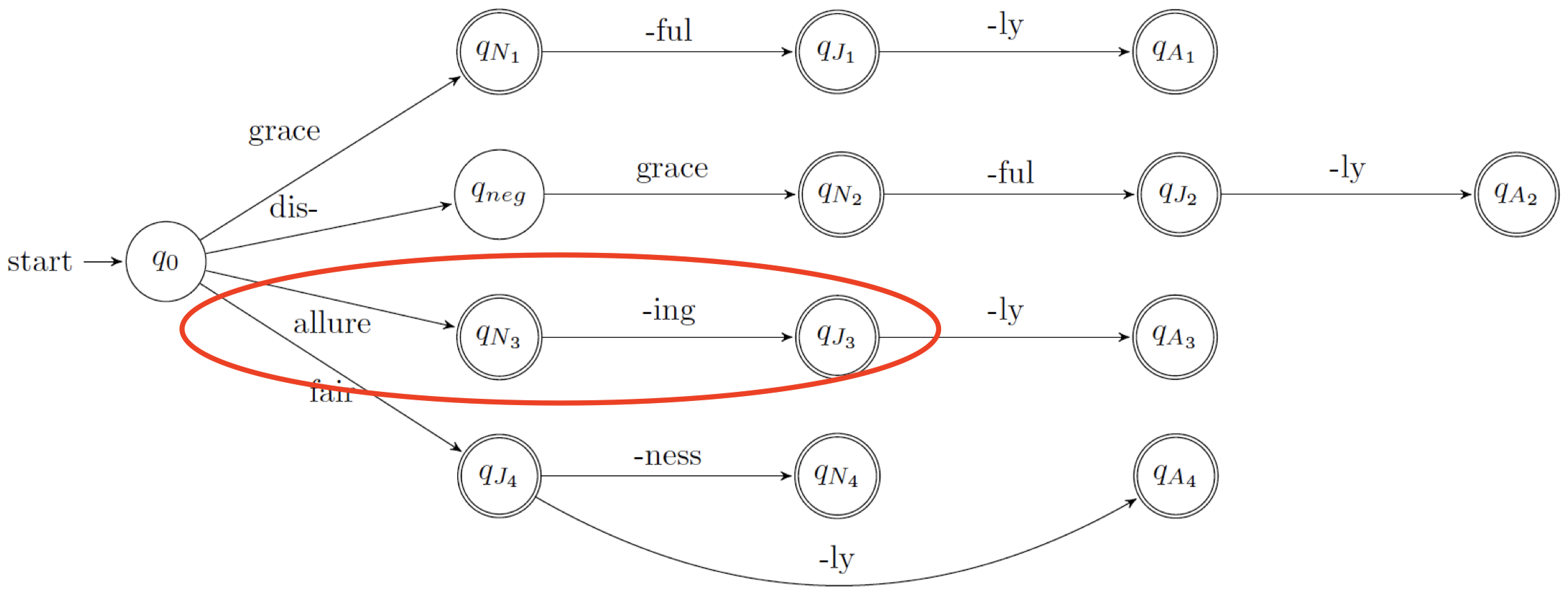• FSA：$\textit{allure}+\textit{ing}=\textit{allureing}$
• FST：$\textit{allure}+\textit{ing}=\textit{alluring}$

• FST 在 FSA 的基础上增加了字符串的输出能力：
• 包含一个 输出字母集（output alphabet）
• 现在，转移函数会采用输入符号并发出输出符号 $(Q,\Sigma, \Sigma, Q)$
其中，第一个 $Q$ 表示输入状态，最后一个 $Q$ 表示输出状态，第一个 $\Sigma$ 表示输入字母集，第二个 $\Sigma$ 表示输出字母集。
• 可以进行 加权 $=$ WFST
• 为转移函数进行记分
• 例如：将 编辑距离（edit distance）作为 WFST，它接受一个输入字符串和一个输出字符串，并返回从输入字符串转变为输出字符串的代价。
• 只有当两个字符串相同时，代价才为 0。

### 5.2 编辑距离自动机

\begin{align} \delta(q,a,a,q) &= \delta(q,b,b,q)=0 \\ \delta(q,a,b,q) &= \delta(q,b,a,q)=1 \\ \delta(q,a,\varepsilon,q) &= \delta(q,b,\varepsilon,q)=1 \\ \delta(q,\varepsilon,a,q) &= \delta(q,\varepsilon,b,q)=1 \end{align}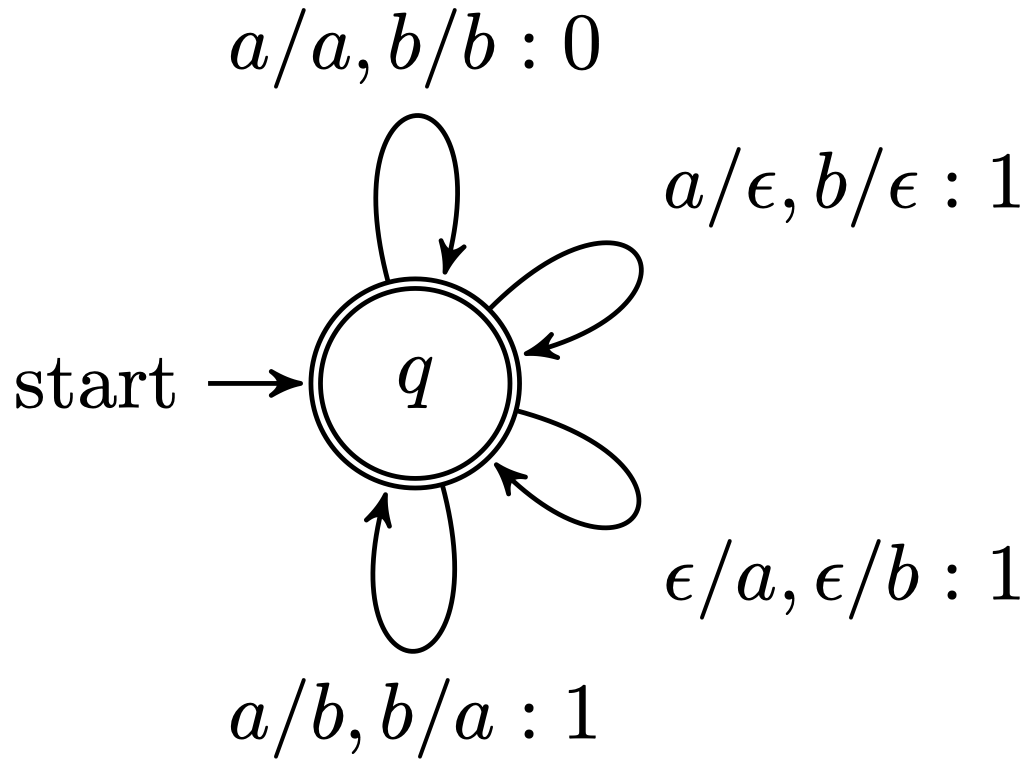• 输入字符串为 $ab$，输出字符串为 $bb$：
$ab \to bb: 1$
• 输入字符串为 $ab$，输出字符串为 $aaab$：
$ab \to aaab: 2$

### 5.3 FST 用于屈折形态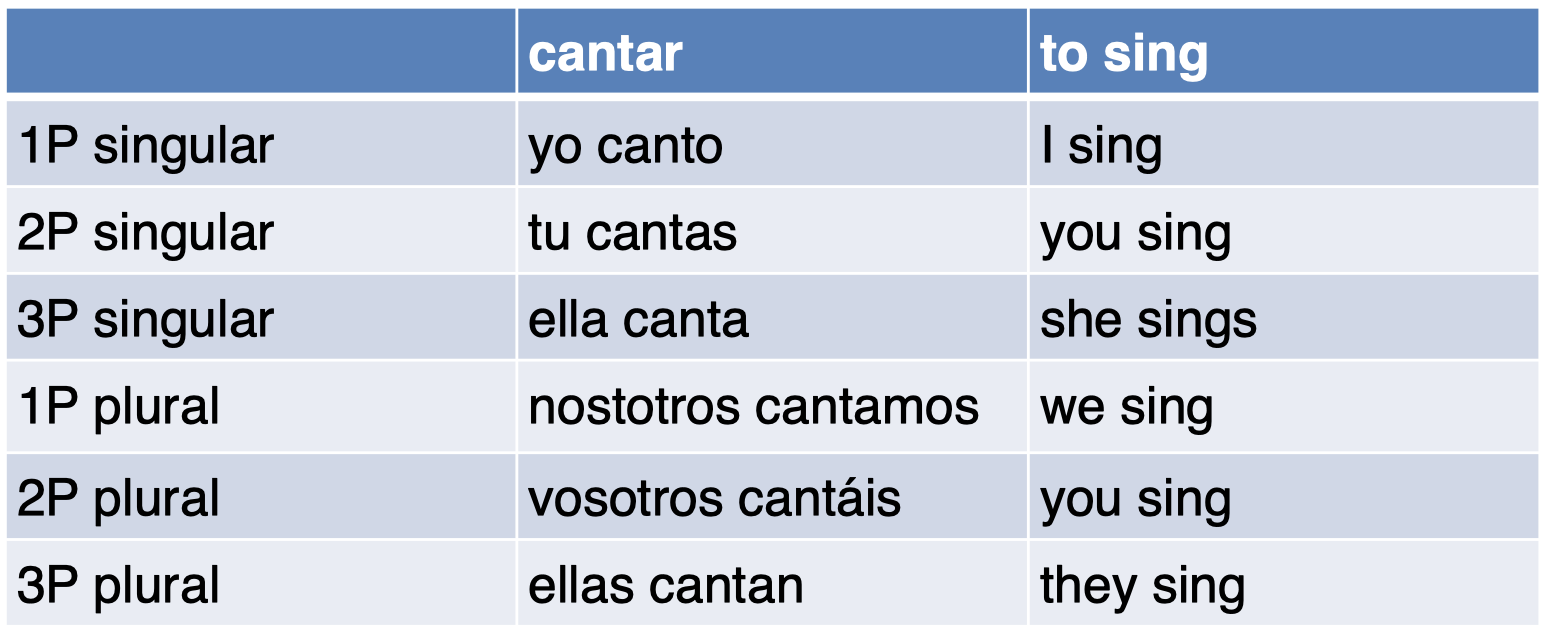$\textit{canto}\to \textit{cantar} + \text{VERB} + \text{present} + 1\text{P} + \text{singular}$

### 5.4 FST 用于西班牙语屈折形态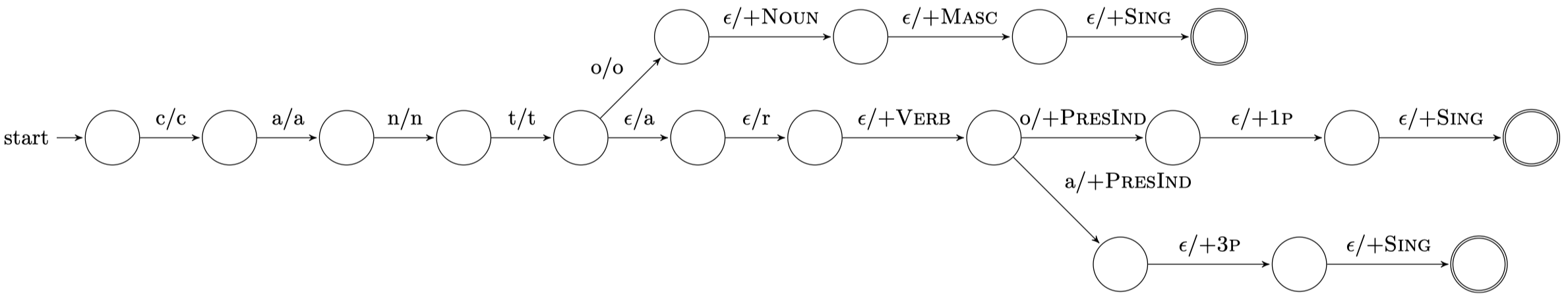• $\textit{canto} \to \textit{canto} + \text{Noun} + \text{Masc} + \text{Sing}$
• $\textit{canto}\to \textit{cantar} + \text{Verb} + \text{PresInd} + 1\text{P} + \text{Sing}$

### 5.5 FST 压缩

• 记为 $T_1\circ T_2$，压缩两个 FST 会产生一个新的 FST
• 另外，我们也可以将 FST 和 FSA 进行压缩，结果为一个新的 FST

• 在英语中，-$ed$ 用于单数过去时态
• $\textit{cook}\to \textit{cooked}$
• $\textit{want}\to \textit{wanted}$
• 但是，当单词以 “$e$” 结尾时，我们希望避免产生连续的 “$e$”（例如：$\textit{bakeed}$）
• 解决方案：构建 2 个 FST
• FST $T_1$：$\textit{bake}+\text{PAST}\to \textit{bake}+\textit{ed}$
• FST $T_2$：$\textit{bake}+\textit{ed}\to \textit{baked}$

## 6. 自然语言是正则语言吗？

### 6.1 正则语言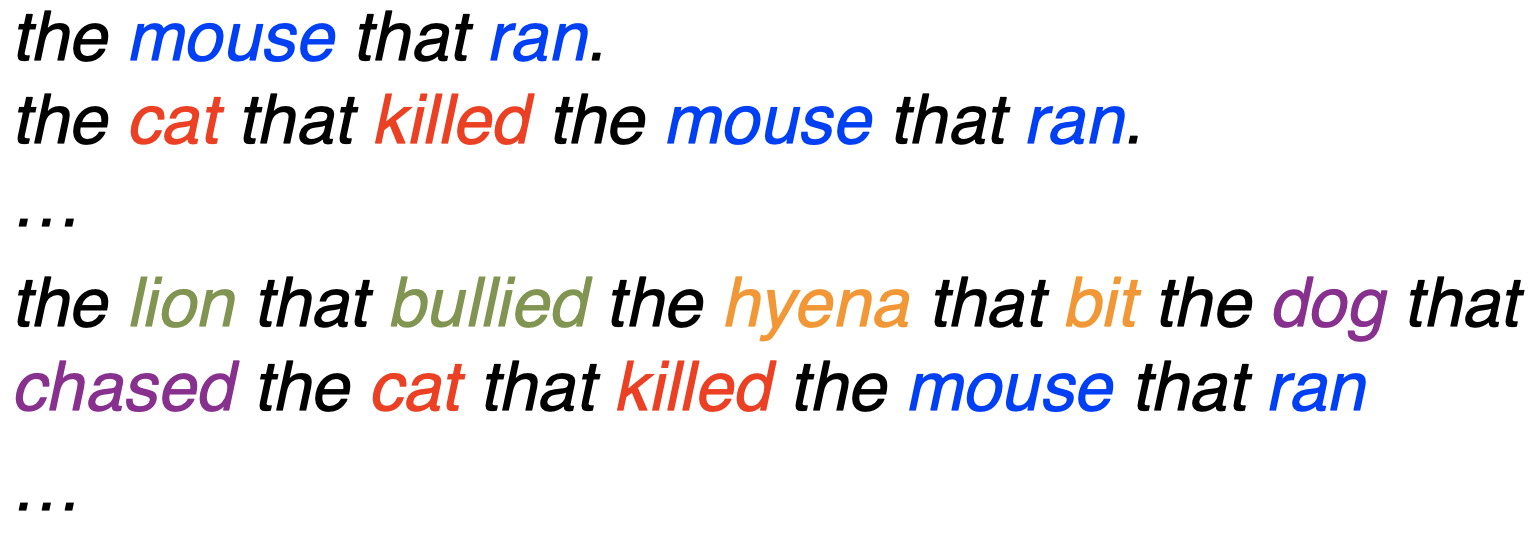• 句子长度是无界的（递归的），但是结构是 局部的 $\to$ 可以采用 FSA 描述 $=$ 正则语言。
• $(\text{Det Noun Prep Verb})^*$

### 6.2 非正则语言

• $\color{orange}{(} a+\color{blue}{(}b\times \color{red}{(} c\,/\,d \color{red}{)} \color{blue}{)} \color{orange}{)}$
• 算术表达式可以具有任意多的开放圆括号。
• 需要记住出现了多少个开放圆括号，以产生相同数量的闭合圆括号。
• 无法用有限数量的状态描述。

### 6.3 中心嵌入

• $\textit{The cat loves Mozart}$
• $\textit{The cat }\color{red}{\textit{the dog chased }}\textit{loves Mozart}$
• $\textit{The cat }\color{red}{\textit{the dog }}\color{blue}{\textit{the rat bit }}\color{red}{\textit{chased }}\textit{loves Mozart}$
• $\textit{The cat }\color{red}{\textit{the dog }}\color{blue}{\textit{the rat }}\color{orange}{\textit{the elephant admired }}\color{blue}{\textit{bit }}\color{red}{\textit{chased }}\textit{loves Mozart}$

## 7. 总结

• 在形式语言理论的框架下，语言和语法的概念
• 正则语言
• 有限状态自动机：接收器和转换器
• 封闭性质
• 加权变体 & 最短路径推断
• 将 FSA/FST 应用于编辑距离和单词形态学

## 8. 扩展阅读

• E18, Chapter 9.1本作品采用知识共享署名-非商业性使用-相同方式共享 4.0 国际许可协议进行许可。 欢迎转载，并请注明来自：YEY 的博客 同时保持文章内容的完整和以上声明信息！